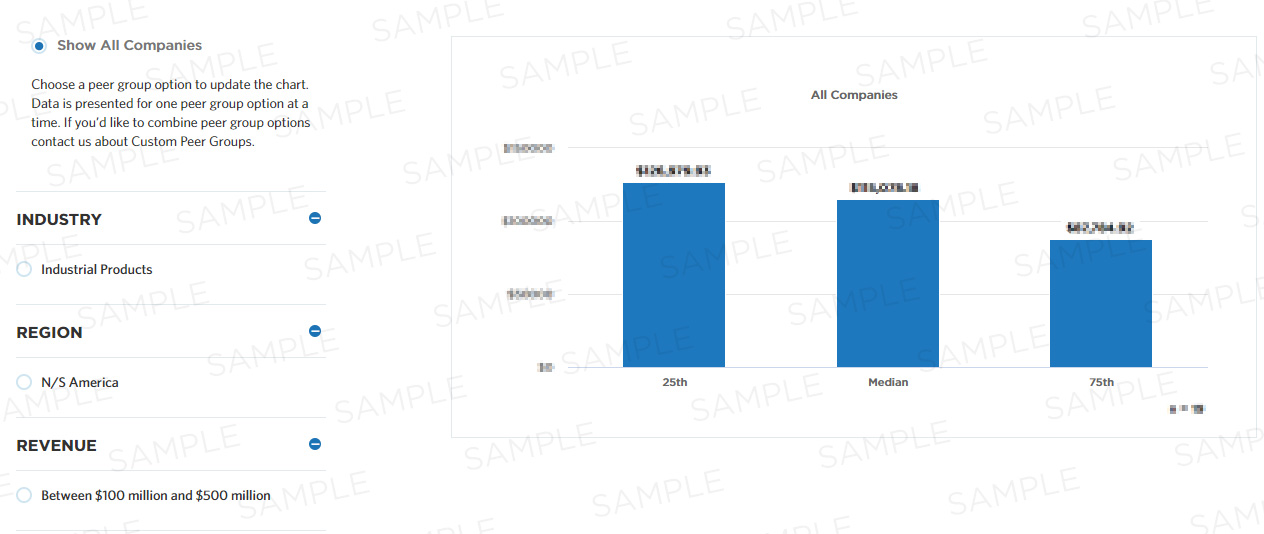# Percentage error for the inventory cost forecast

This measure calculates the inventory cost forecasting percentage error. It is calculated as the absolute value of ((Projected – Actual inventory cost) / Actual inventory cost) * 100.

## Benchmark Data

Measure Category:
Process Efficiency
Measure Id:
101760
Total Sample Size:
2,804 All Companies
Performers:
25th
Median
75th
Key Performance
Indicator:
No## Compute this Measure

Units for this measure are percent.

`Percentage error for the inventory cost forecast`

## Key Terms

#### Process efficiency

Process efficiency represents how well a process converts its inputs into outputs. A process that converts 100% of the inputs into outputs without waste is more efficient than one that converts a similar amount of inputs into fewer outputs.